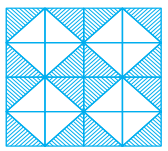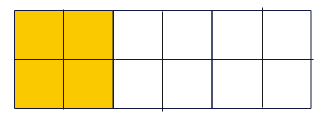# Important Questions for Class 6 Maths Ratio and Proportion

In this page we have Important Questions for Class 6 Maths Ratio and Proportion . Hope you like them and do not forget to like , social share and comment at the end of the page.

Question 1

Find the ratio of the following:
(a) 63 to 45
(b) 256 to 512
(c) 66 km to 121 km
(d) 3 minutes to 45 minutes
(e) ½ hour to 45 minutes
(f) 1cm to 10 mm
(g) 45 paise to Rs 1
(h) 100 mL to 2 litre

(a) 63: 45= 7:6
(b) 256 : 512= 1: 2
(c) 66 km : 121 km= 6: 11
(d) 3 minutes : 45 minutes=1 : 15
(e) ½ hour to 45 minutes= 30 min to 45 minutes= 2 :3
(f) 1cm to 10 mm=10 mm to 10 mm=1:1
(g) 45 paise to Rs 1= 45 paise to 100 paise=9 : 20
(h) 100 mL to 2 litre= 100 mL to 2000ml= 1:20

Question 2
In a School, out of 1200 students, 300 are girls. Find the ratio of
(a) Number of girls to the total number of students.
(b) Number of boys to the number of girls.
(c) Number of boys to the total number of students.

(a) 300: 1200 =1:4
(b) Number of boys=1200-300=900
Hence Ratio = 900/300= 3:1
(c) Ratio = 900/1200= 3:4

Question 3
Cost of a dozen bananas is Rs 180 and cost of 8 lemons is Rs 56. Find the ratio of the cost of a banana to the cost of a lemon.

Cost of 12 banana is Rs 180
Cost of 1 Banana is Rs 180/12 = Rs15
cost of 8 lemons is Rs 56.
Cost of 1 lemon is Rs 56/8 =Rs 7
ratio of the cost of a banana to the cost of a lemon = 15: 7

Question 4
Determine if the following are in proportion.
(a) 25, 75, 80, 240
(b) 11, 121, 9, 99
(c) 12, 144, 25, 625
(d) 32, 48, 70, 210
(e) 4, 6, 8, 12
(f) 33, 44, 75, 100

(a)25, 75, 80, 240
25: 75= 1: 3
80: 240 = 1:3
Therefore
25: 75::80: 240
(b)11, 121, 9, 99
11: 121= 1: 11
9: 99 = 1:11
Therefore
11: 121::9: 99
(c)12, 144, 25, 625
12:144= 1:12
25:625= 1:25
So not in proportion
(d)32, 48, 70, 210
32:48= 2:3
70:210=1:3
32, 48, 70, 210
(e)4, 6, 8, 12
4: 6=2:3
8: 12=2:3
Therefore
4: 6::8:12
(f) 33, 44, 75, 100
33:44= 3:4
75:100= 3:4
Therefore
33:44::75:100

Question 6
Determine if the following ratios form a proportion. Also, write the middle terms and extreme terms where the ratios form a proportion.
(a) 10 cm: 1 m and Rs 10: Rs 100
(b) 39 litres: 65 litres and 6 bottles: 10 bottles
(c) 2 kg: 50 kg and 25 g: 625 g
(d) 200 mL: 2.5 litre and Rs 4: Rs 50

(a)10 cm: 1 m and Rs 10: Rs 100
10 cm: 1 m= 1: 10
Rs 10: Rs 100 = 1:10
Therefore it is in proportion
10 cm: 1 m :: Rs 10: Rs 100
Middle Terms: 1 m and Rs 10
Extreme Terms : 10 cm and Rs 100
(b)39 litres: 65 litres and 6 bottles: 10 bottles
39 litres: 65 litres= 3: 5
6 bottles: 10 bottles = 3: 5
Therefore
39 litres: 65 litres ::6 bottles: 10 bottles
Middle Terms: 65 litres and 6 bottles
Extreme Terms : 39 litres and 10 bottles
(c)2 kg: 50 kg and 25 g: 625 g
2 kg: 50 kg = 1:25
25 g: 625 g= 1:25
Therefore
2 kg: 50 kg :: 25 g: 625 g
Middle Terms: 50 kg and 25 g
Extreme Terms : 2 kg and 625 g
(d)200 mL: 2.5 litre and Rs 4: Rs 50
Rs 4: Rs 50= 2: 25
Therefore
200 mL: 2.5 litre :: Rs 4: Rs 50
Middle Terms: 2.5 litre amd Rs 4
Extreme Terms : 200 mL and Rs 50

Question 7
Aakash earns Rs 20000 in 10 days. How much will she earn in 30 days?

Earning of 10 days is Rs 20000
Earning of 1 days is Rs 20000/10=Rs 2000
Earning of 30 days is Rs 2000 * 30= Rs60000

Question 8
The weight of 72 books is 144 kg. What is the weight of 10 such books?

weight of 72 books is 144 kg
weight of 1 books is 144/72=2 kg
weight of 10 books is 2 kg * = 20kg

Question 9
find the ratio of the area of the shaded portion to that of the whole figure
(i)(ii)(i)we have 32 part of same right angle triangle. 16 are shaded
So Ratio of the area of the shaded portion to that of the whole figure= 16/32= 1: 2
(ii) we have 12 small squares and 4 are shaded So Ratio of the area of the shaded portion to that of the whole figure= 4/12= 1: 3### Practice Question

Question 1 What is $\frac {1}{2} + \frac {3}{4}$ ?
A)$\frac {5}{4}$
B)$\frac {1}{4}$
C)$1$
D)$\frac {4}{5}$
Question 2 Pinhole camera produces an ?
A)An erect and small image
B)an Inverted and small image
C)An inverted and enlarged image
D)None of the above